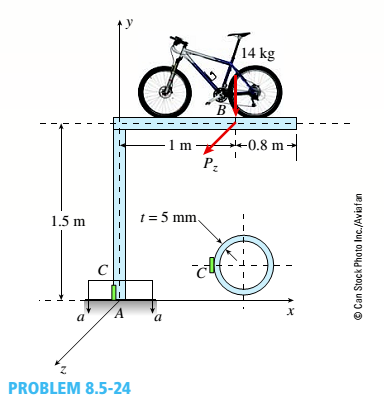# Repeat Problem 8.5-22 but replace the square tube column with a circular tube having a wall thickness r = 5 mm and the same cross-sectional area (3900 mm 2 ) as that of the square tube in figure b in Problem 8.5-22. Also, add force P. = 120 N at B (a) Find the state of plane stress at C. (b) Find maximum normal stresses and show them on a sketch of a properly oriented element. (c) Find maximum shear stresses and show them on a sketch of a properly oriented element.### Mechanics of Materials (MindTap Co...

9th Edition
Barry J. Goodno + 1 other
Publisher: Cengage Learning
ISBN: 9781337093347

#### Solutions

Chapter
Section### Mechanics of Materials (MindTap Co...

9th Edition
Barry J. Goodno + 1 other
Publisher: Cengage Learning
ISBN: 9781337093347
Chapter 8, Problem 8.5.24P
Textbook Problem
3 views

## Repeat Problem 8.5-22 but replace the square tube column with a circular tube having a wall thickness r = 5 mm and the same cross-sectional area (3900 mm2) as that of the square tube in figure b in Problem 8.5-22. Also, add force P. = 120 N at B(a) Find the state of plane stress at C. (b) Find maximum normal stresses and show them on a sketch of a properly oriented element. (c) Find maximum shear stresses and show them on a sketch of a properly oriented element.(a)

To determine

The state of plane stress on an element C.

### Explanation of Solution

Given information:

The cross-section of the bicycle rack tubing is circular.

The weight of the bicycle = 14 kg is represented as point load applied at B on a plane frame model of the rack.

A force in z direction has also been added Pz= 120 N

Weight density of the steel γ=77kN/m3

Calculation:

From the figure, let us consider horizontal portion of the cycle rack. The weight of the cycle is considered as the point load and this load produces a moment and a direct compressive load in the beam along Y-direction.

Also, a load Pzhas been added in z direction. This load will produce bending moment in the horizontal beam and twisting moment in the vertical portion of the beam.

The cross-sectional area of the beam is given by

A=3900mm2=3.9×103m2

A=π4(d2(dt)2)3900=π4(d2(d5)2)

d=499.06mm

The moment in the vertical beam =

M=14×9.81×1M=137.34Nm

The moment in the horizontal beam due to load Pz= Mz=Pz×1m

Mz=120×1=120Nm

Total moment Me=137.34+120=257.34Nm

The load Pzwill produce twisting moment equal to Mz. This twisting moment will produce the shear stress at the base of rack.

From the properties of the cross-section, the moment of inertia is

I=πd464π(dt)464

I=π×(499

(b)

To determine

The maximum normal stress and the sketch of properly oriented element.

(c)

To determine

The maximum shear stress and the sketch of properly oriented element.

### Still sussing out bartleby?

Check out a sample textbook solution.

See a sample solution

#### The Solution to Your Study Problems

Bartleby provides explanations to thousands of textbook problems written by our experts, many with advanced degrees!

Get Started

Find more solutions based on key concepts
What are the major components of a building?

Engineering Fundamentals: An Introduction to Engineering (MindTap Course List)

If the couple applied to the steering wheel is to have the magnitude C=120lbin., determine F.

International Edition---engineering Mechanics: Statics, 4th Edition

Why would a manager prefer a decision tree instead of a decision table?

Systems Analysis and Design (Shelly Cashman Series) (MindTap Course List)

Describe ways to use voice command personal assistant apps.

Enhanced Discovering Computers 2017 (Shelly Cashman Series) (MindTap Course List)

How does an injector-type mixing chamber work?

Welding: Principles and Applications (MindTap Course List)

If your motherboard supports ECC DDR3 memory, can you substitute non-ECC DDR3 memory?

A+ Guide to Hardware (Standalone Book) (MindTap Course List)